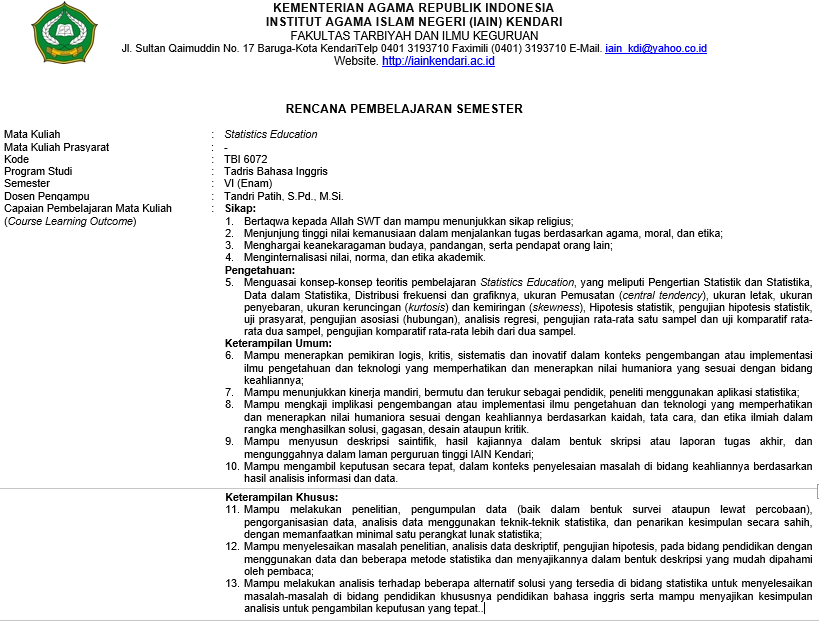### TBI 6072-A-20202 STATISTICS EDUCATION (Kelas A)

Students are expected to be able to master the theoretical concepts of Statistics Education learning, which include Understanding Statistics and Statistics, Data in Statistics, Frequency Distribution and its graphs, central tendency, size of location, size of dispersion, size of kurtosis and skewness, Statistical hypothesis, statistical hypothesis testing, assumption test, association (relationship) test, regression analysis, one-sample test of mean and two independent sample comparative t-test, comparative test for the mean of more than two samples### TBI 6072-B-20202 STATISTICS EDUCATION (Kelas B)

Students are expected to be able to master the theoretical concepts of Statistics Education learning, which include Understanding Statistics and Statistics, Data in Statistics, Frequency Distribution and its graphs, central tendency, size of location, size of dispersion, size of kurtosis and skewness, Statistical hypothesis, statistical hypothesis testing, assumption test, association (relationship) test, regression analysis, one-sample test of mean and two independent sample comparative t-test, comparative test for the mean of more than two samples